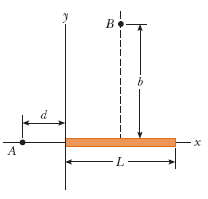# Question A rod of length L lies along the x axis with its left end at the origin. It has a nonuniform charge density λ = αx, where α is a positive constant. Calculate the electric potential at point B , which lies on the perpendicular bisector of the rod a distance b above the x axis. (Use the following as necessary: α, ke, L, b, and d.)